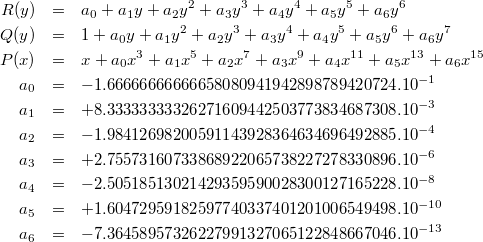Version 3 (modified by sam, 9 years ago) (diff)

--

# Trigonometric functions

Our research notes about implementation of fast trigonometric functions.

## Minimax polynomial for sin(x)

### Absolute error

Suppose we want to approximate sin(x) on [-π/2; π/2] with a polynomial P(x) such that the absolute error is never more than E: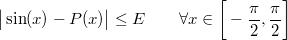We know sin(x) is an odd function, so instead we look for a polynomial Q(x) such that P(x) = xQ(x²):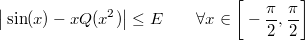Substitute y for x² and reduce the range to positive values: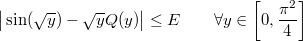Divide through by √y:If we want to force the asymptotic behaviour in x=0, we substitute Q(y) with 1+yR(y):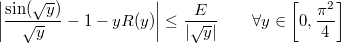Divide through by y: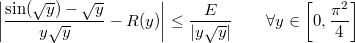We then use the following code:

static real myfun(real const &x)
{
real y = sqrt(x);
return (sin(y) - y) / (x * y);
}

static real myerr(real const &x)
{
return re(x * sqrt(x));
}

RemezSolver<6> solver;
solver.Run(real::R_1 >> 400, real::R_PI_2 * real::R_PI_2, myfun, myerr, 15);


These are the resulting R, Q and P: